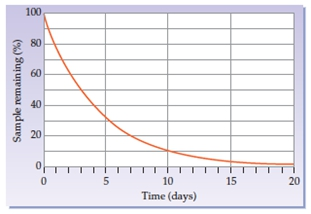Start typing, then use the up and down arrows to select an option from the list.# GOB Chemistry

Learn the toughest concepts covered in your GOB - General, Organic, and Biological Chemistry class with step-by-step video tutorials and practice problems.

Nuclear Chemistry

Half-Life is the amount of time required for half of a radioisotope to decay.

1

#### concept2m
Play a video:
here, we're going to say that half life, which uses the variable T sub half is the amount of time required for half Or 50% of a radio isotope to decay. Now, radioisotope is just the isotopic version of an element that has an unstable nucleus and emits radiation as a decades here are going to say that the radioactive the kid, an unknown radioisotope with a half life of one day is displayed, asked. So here in half life is one day, which means that after one day, half of my substance will be gone. So let's say that we're starting out with some unknown isotope. Remember isotope, your ex is the symbol A is a mass number and Z is the atomic number. Let's say that we're starting out with 10 grands of it. Nothing has happened yet. So 0/2 lives have occurred. So initially I'm starting out with 100% of my substance. Now one day is allowed to pass. And remember here a half life is equal to a day. Now half lives are not always one day, they vary based on the element. So after one day I've lost half of my substance. So now I only have five grand's left, So 1/2 life is passed and all I have left is 50%. I've lost half of what I had another day passes, so I've lost another half. So I had five to begin with. Another day passes. So now I have only half of that left, so 2.5. That means two half lives have passed so far, and I only have 25% remaining. Remember 100% was 10 g, and I only have 2.5 g And then one more half life passes. So now I'm losing another half of this, 2.5, Leaving me with 1.25. So three life, three half lives have passed, and now I only have 12.5% remaining of the original amount I started with. So this is how half life works and to keep going and going and going, I can go to 1/4 half life. Why would lose another half of this? Okay, so that's the way you have to understand half life. It's the amount of time it takes to lose half or 50% of my starting material.
2

#### concept1m
Play a video:
with half. Like we can also determine the amount of radio isotope remaining. He was going to say the fraction percentage and final amount of radioisotope after each half life can be calculated by different means. Alright, so first we have our fraction formula. This just tells us the fraction remaining of my initial amount Here. That would just be .5 to the end. The .5 comes from the fact that a half life is a half And when you punch a half into your calculator gives you back .5 end is just the number of half lives. One important thing to remember about this is if you also also multiply the fraction remaining value by 100%, that will give you the percentage remaining of the radio isotopes. So keep that in mind. You can calculate either the fraction remaining or the percentage remaining. Now we also have our final amount formula. This tells me how much of my final amount is actual remaining. Not a fraction of a percent but an actual amount. Let's say. You started out with 100 g of a substance. You can use this formula to tell you how much of that 100 g is remaining. So here this would just be brackets with I this would be your initial amount Times what we just saw, our fractional remaining, our fraction remaining, which is .5 to the end. So you can use either formula if you're trying to find either the fraction percentage or final amount remaining for radio isotope.
3

#### example1m
Play a video:
Here, we're told that sodium 20 for a radioisotope used in examining human circulation has a half life of about 15 days, approximately what percentage of a sample remain after three months. If a month is considered 30 days. All right. So, they're asking for the percentage remaining. Remember? The percentage remaining is just your fraction remaining, so .5 to the end Times 100%. Here, we have to figure out how many half lives have occurred. So here we're going to say three months have passed and each month is 30 days. So that's three times 30. So that's a total of 90 days have occurred. Each half life is 15 days. So, if I do 90 divided by 15, it gives me back. six. That is the number of half lives that have occurred. So then all we do here is we're gonna say five To the six times Equals 15625%. This is the percentage remaining of my radio isotope.
4
Problem

The half-life of arsenic-74 is about 18 days. If a sample initially contains 100.00 mg arsenic-74, what mass (in mg) would be left after 72 days?

5
Problem

The half-life of iodine-131, an isotope used in thyroid therapy, is 8.021 days. What percentage of iodine-131 remains in a sample that is estimated to be 40.11 days old?

6
Problem

What is the concentration of a CO2 in a container after 4 half-lives if 0.325 mol of CO2 is initially placed into a 5.0 L reaction vessel?

7
Problem

What is the half-life of the radioisotope that shows the following plot of remaining percentage vs. time?